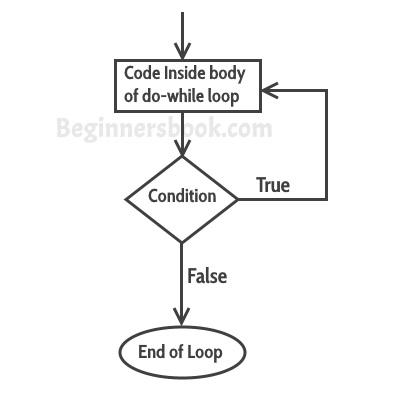### Do while loop in C++

As discussed in the last article about while loop, a loop is used for repeating a block of statements until the given loop condition returns false. In this article we will see do-while loop. do-while loop is similar to while loop, however there is a difference between them: In while loop, condition is evaluated first and then the statements inside loop body gets executed, on the other hand in do-while loop, statements inside do-while gets executed first and then the condition is evaluated.

#### Syntax of do-while loop

```do
{
statement(s);
} while(condition);```

## How do-while loop works?

First, the statements inside loop execute and then the condition gets evaluated, if the condition returns true then the control jumps to the “do” for further repeated execution of it, this happens repeatedly until the condition returns false. Once condition returns false control jumps to the next statement in the program after do-while.## do-while loop example in C++

```#include <iostream>
using namespace std;
int main(){
int num=1;
do{
cout<<"Value of num: "<<num<<endl;
num++;
}while(num<=6);
return 0;
}```
Output:
```Value of num: 1
Value of num: 2
Value of num: 3
Value of num: 4
Value of num: 5
Value of num: 6```

## Example: Displaying array elements using do-while loop

Here we have an integer array which has four elements. We are displaying the elements of it using do-while loop.
```#include <iostream>
using namespace std;
int main(){
int arr[]={21,99,15,109};
/* Array index starts with 0, which
* means the first element of array
* is at index 0, arr
*/
int i=0;
do{
cout<<arr[i]<<endl;
i++;
}while(i<4);
return 0;
}```
Output:
```21
99
15
109```